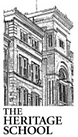## Math

###### Algebra I

This course provides a formal development of the algebraic skills and concepts necessary for students to succeed in advanced courses.  In particular, the instructional program in this course provides for the use of algebraic skills in a wide range of problem-solving situations.  The concept of function is emphasized throughout the course.  Topics include: (1) operations with real numbers, (2) linear equations and inequalities, (3) relations and functions, (4) polynomials, (5) algebraic fractions, and (6) nonlinear equations. Students who complete Algebra I would be taking Geometry next.

###### Geometry

This course is designed to emphasize the study of the properties and applications of common geometric figures in two and three dimensions.  It includes the study of transformations and right triangle trigonometry.  Inductive and deductive thinking skills are used in problem solving situations, and applications to the real world are stressed.  It also emphasizes writing proofs to solve (prove) properties of geometric figures.  Students who complete Geometry should take Algebra II next.

###### Algebra II

This course is designed to build on algebraic and geometric concepts.  It develops advanced algebra skills such as systems of equations, advanced polynomials, imaginary and complex numbers, quadratics, and concepts and includes the study of trigonometric functions.  It also introduces matrices and their properties.  The content of this course are important for students’ success on both the ACT and college mathematics entrance exams.  Students who complete Algebra II should take Pre-Calculus next.